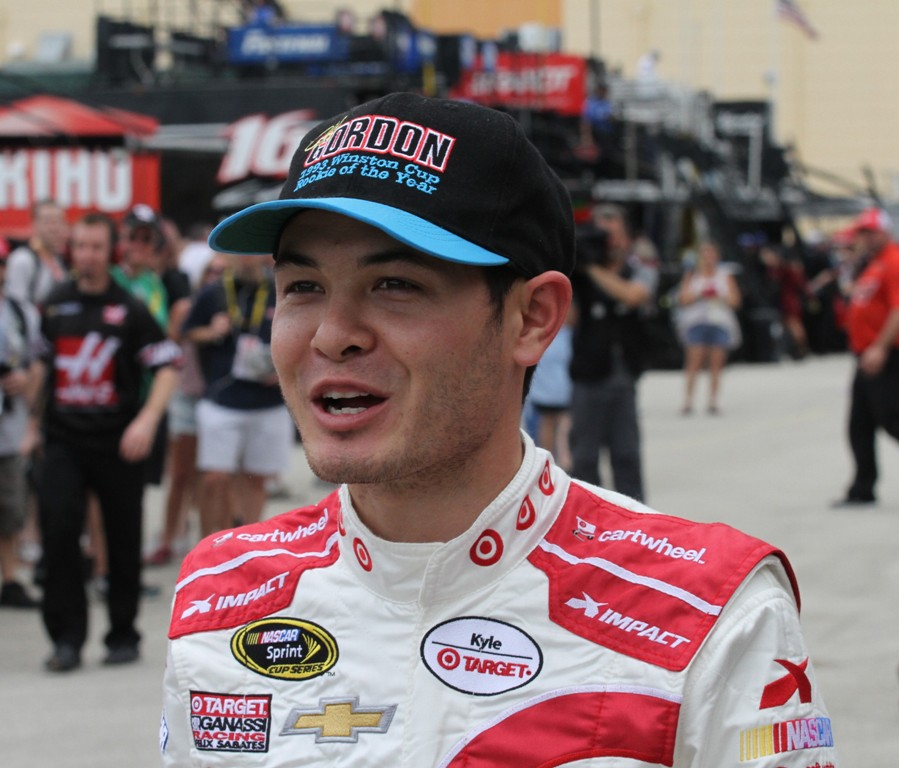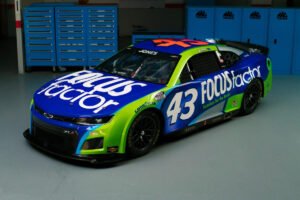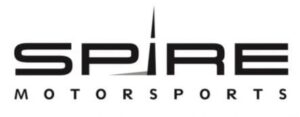December 1, 2021

# OnPitRoad.com Pick ‘Em Fantasy Pick Results for Ford EcoBoost 300 at Homestead-Miami SpeedwayThe Picks

Ronald Costigan and Ashley McCubbin picked Chase Elliott, Erik Jones and Kyle Busch. Elliott finished eighth with Jones third and Busch 30th. Points: 36+42+15=93

Justin Tucker picked Erik Jones, Ryan Blaney and Kyle Busch. Blaney finished fifth. Points: 42+39+15=96

Robert Hill picked Darrell Wallace Jr., Erik Jones and Ryan Sieg. Wallace finished 10th and Sieg 14th. Points: 34+42+30=106

Tim Johnston, Marshall Gabell and Brooke Clarke picked Chase Elliott, Ryan Blaney and Ben Rhodes. Rhodes finished 20th. Points: 36+39+24=99

Kreistina Blinova picked Ty Dillon, Austin Dillon and Ben Rhodes. Ty finished seventh with Austin second. Points: 37+43+24=104

Kyle Magda picked Derek White, Ryan Blaney and Daniel Suarez. White finished 35th with Suarez sixth. Points: 9+39+38=86

Steve Love picked Regan Smith, Kyle Larson and Kyle Busch. Smith finished ninth with Larson won. Points: 35+48+15=98

Jeni Harris picked Regan Smith, Chase Elliott and Erik Jones. Points: 35+36+42=113

Doug Townsend picked Ryan Blaney, Kyle Busch and Chris Buescher. Buescher finished 11th. Points: 39+15+33=87

Brandon Feeney picked Chase Elliott, Kyle Busch and Chris Buescher. Points: 36+15+33=84

Rory Townsend picked Chase Elliott, TJ Bell and Ben Rhodes. Bell finished 39th. Points: 36+5+24=65

Parker Ledger picked Regan Smith, Chase Elliott and Ben Rhodes. Points: 35+36+24=95

Alex George picked Chase Elliott, Kyle Larson and Ben Rhodes. Points: 36+48+24=108

Mike Cousins picked Ty Dillon, Chris Buescher and Ben Rhodes. Points: 37+33+24=94

Jeffrey Breazeale picked Regan Smith, Ryan Blaney and Kyle Busch. Points: 35+39+15=89

Stephen Placido picked Darrell Wallace Jr., Ryan Blaney and Ben Rhodes. Points: 34+39+24=97

The Results

1. Jeni Harris – 113
2. Alex George – 108
3. Robert Hill – 106
4. Kreistina Blinova – 104
5. Tim Johnston, Marshall Gabell and Brooke Clarke – 99
6. Steve Love – 98
7. Stephen Placido – 97
8. Justin Tucker – 96
9. Parker Ledger – 95
10. Mike Cousins – 94
11. Ronald Costigan and Ashley McCubbin – 93
12. Jeffrey Breazeale – 89
13. Doug Townsend – 87
14. Kyle Magda – 86
15. Brandon Feeney – 84
16. Rory Townsend – 65

Current Fall XFINITY Series Standings

1. (Points Leader Post Phoenix) Justin Tucker – 822+96=918
2. Steve Love – 814+98=912 (-6)
3. Jeffrey Breazeale – 813+89=902 (-16)
4. Ronald Costigan – 792+93=885 (-33)
5. Brandon Feeney – 797+84=881 (-37)
6. Doug Townsend – 789+87=876 (-42)
7. Ashley McCubbin – 779+93=872 (-46)
8. Jeni Harris – 752+113=865 (-53)
9. Robert Hill – 738+106=844 (-74)
10. Kreistina Blinova – 735+104=839 (-79)
11. Tim Johnston, Marshall Gabell and Brooke Clarke – 738+99=837 (-81)
12. Parker Ledger – 742+95=837 (-81)
13. Stephen Placido – 717+97=814
14. Mike Cousins – 719+94=813
15. Alex George – 656+108=764
16. Kyle Magda – 606+86=692
17. Rory Townsend – 543+65=608

CONGRATULATIONS to JUSTIN TUCKER on winning the 2015 ONPITROAD.COM FALL FANTASY PICK ‘EM NASCAR CAMPING WORLD TRUCK SERIES CHAMPIONSHIP. Justin scored the championship six points ahead of Steve Love.

PREVIOUS SEASONAL CHAMPIONS INCLUDE: Ashley McCubbin (Spring 2014, Summer 2014 and Summer 2015) and Justin Tucker (Fall 2014 and Spring 2015).

Full Season XFINITY Series Standings

2. Ronald Costigan – 3648+93=3741 (-73)
3. Jeffrey Breazeale – 3620+89=3709
4. Ashley McCubbin – 3596+93=3689
5. Brandon Feeney – 3559+84=3643
6. Doug Townsend – 3533+87=3620
7. Steve Love – 3513+98=3611
8. Brooke Clarke – 3410+99=3509
9. Tim Johnston – 3402+99=3501
10. Stephen Placido – 3391+97=3488
11. Kyle Magda – 3313+86=3399
12. Mike Cousins – 3295+94=3389
13. Parker Ledger – 3286+95=3381
14. Marshall Gabell – 3280+99=3379
15. Kreistina Blinova – 3268+104=3372
16. Jeni Harris – 3219+113=3332
17. Robert Hill – 3218+106=3324
18. Alex George – 3060+108=3168
19. Rory Townsend – 2866+65=2931

CONGRATULATIONS to JUSTIN TUCKER on winning the 2015 ONPTIROAD.COM CAMPING WORLD TRUCK SERIES CHAMPIONSHIP. Justin scored the championship, 73 points ahead of Ronald Costigan. The 2015 season marks the first time that ONPITROAD.com has crowned a full-season champion.

Fall Overall Standings

1. (Points Leader Post Cup Martinsville) Steve Love – 2268+73+98=2439
2. (Points Leader Post Truck Phoenix) Doug Townsend – 2255+87+87=2429 (-10)
3. Brandon Feeney – 2202+81+84=2367 (-72)
4. Justin Tucker – 2173+86+96=2355 (-84)
5. Parker Ledger – 2178+53+95=2326
6. Ashley McCubbin – 2142+86+93=2321
7. Ronald Costigan – 2127+86+93=2306
8. Jeni Harris – 2137+53+113=2303
9. Brooke Clarke – 2113+80+99=2292
10. Kreistina Blinova – 2079+80+104=2263
11. Mike Cousins – 2077+74+94=2245
12. Tim Johnston – 2072+68+99=2239
13. Stephen Placido – 2038+80+97=2215
14. Marshall Gabell – 1992+70+99=2161
15. Aex George – 1965+45+108=2118
16. Jeffrey Breazeale – 1942+71+89=2102
17. Rory Townsend – 1897+66+65=2028
18. Kyle Magda – 1453+70+86=1609
19. Robert Hill – 1711+48+106=1266

Full Season Overall Points Standings

1. (Points Leader Post Cup Kansas) Justin Tucker – 8939+86+96=9121
2. Ronald Costigan – 8758+86+93=8937 (-184)
3. Ashley McCubbin – 8706+86+93=8885
4. Brandon Feeney – 8685+81+84=8850
5. Doug Townsend – 8665+87+87=8839
6. Steve Love – 8604+73+98=8775
7. Stephen Placido – 8471+80+97=8648
8. Parker Ledger – 8465+53+95=8603
9. Brooke Clarke – 8365+80+99=8529
10. Tim Johnston – 8350+68+99=8517
11. Mike Cousins – 8302+74+94=8470
12. Kreistina Blinova – 8230+80+104=8414
13. Jeffrey Breazeale – 8195+71+89=8354
14. Marshall Gabell – 8127+70+99=8296
15. Jeni Harris – 8092+53+113=8258
16. Kyle Magda – 8077+70+86=8233
17. Alex George – 8065+45+108=8218
18. Rory Townsend – 7744+66+65=7875
19. Robert Hill – 7233+48+106=7387

#### You may have missed#### Why Progressive Insurance is the right choice#### FOCUSFACTOR® TO PARTNER WITH RICHARD PETTY MOTORSPORTS#### Spire Motorsports to Field NASCAR Camping World Truck Series Team in 2022